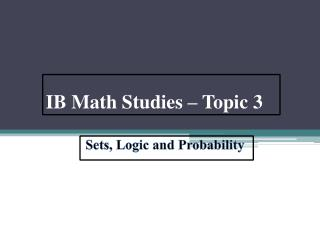# IB Math Studies – Topic 3 - PowerPoint PPT PresentationDownload PresentationIB Math Studies – Topic 3

IB Math Studies – Topic 3Download Presentation## IB Math Studies – Topic 3

- - - - - - - - - - - - - - - - - - - - - - - - - - - E N D - - - - - - - - - - - - - - - - - - - - - - - - - - -
##### Presentation Transcript

1. IB Math Studies – Topic 3 Sets, Logic and Probability

2. IB Course Guide Description

3. IB Course Guide Description

4. Set Theories • A set is any collection of things with a common property: it can be finite. • Example: set of students in a class • If A= {1,2,3,4,5} then A is a set that contains those numbers

5. Subsets • If P & Q are sets then: • P Q means ‘P is a subset of Q’ • In every element in P is also an element in Q Complements • If A contains elements of even numbers then A’ contains elements of odd numbers.

6. Union and Intersection • PQ is the union of sets P and Q meaning all elements which are in P or Q. • PQ is the intersection of P and Q meaning all elements that are in both P and Q. • Example: • A = {1,2,3,4,5} B= {2,4,6,7} AB = {1,2,3,4,5,6,7} AB = {2,4}

7. Venn Diagrams • Venn Diagrams are diagrams used to represent sets of objects, numbers or things. • The universal set is usually represented by a rectangle whereas sets within it are usually represented by circles or ellipses.

8. Sets within Venn Diagram SubsetBA Union AB Intersection AB Disjoint or Mutually Exclusive sets

9. Logic • Proposition • The building block of logic. • This is a statement that can have one of the two value, true or false. • Negation • The negation of a proposition is formed by putting words such as “not” or “do not.” • The negation of a proposition p is “not p” and is written as ¬p. • For example: • p: It is Monday. • ¬p: It is not Monday.

10. Truth Tables • A truth table shows how the values of a set of propositions affect the values of other propositions. • A truth table for negation

11. Compound Propositions • Conjunction:The word and can be used to join two propositions together. Its symbol is . • Disjunction: The word or can also be used to join propositions. Its symbol is . • Exclusive Disjunction:Is true when only one of the propositions is true. • This symbol means that its either p or q but not both.

12. Conjunction/Disjunction and Venn Diagrams

13. Tautology • A tautology is a compound proposition that is always true, whatever the values of the original propositions. • Example: • When all the final entries are ‘T’ the proposition is a Tautology.

14. Contradiction • A Contradiction is a compound proposition that is always false regardless of the truth values of the individual propositions. • Example: • When all the final entries are ‘F’ the proposition is a Contradiction.

15. Logically Equivalent • If two statements have the same truth tables then they are true and false under the exact same conditions. • The symbol for this is • The wording would be said as: “if and only if”

16. Implication • If two propositions can be linked with “If…, then…”, then we have an implication. • p is the antecedent and q is the consequent • The symbol would be  • For example: • P: You steal • Q: you go to prison Therefore, the words “If” and “then” are added. “if you steal, then you go to prison.”

17. Converse, Inverse, and Contrapositive • Converse: q  p • Inverse: p  q • Contrapositive: q p • For example: • P: It is raining • Q: I will get wet • Converse: “If it is raining, then I will get wet.” • Inverse: “It itisn’t raining, then I won’t get wet.” • Contrapositive: “It I’m not wet, then it isn’t raining.”

18. Probability • Probability is the study of the chance of events happening

19. Combined Events • The probability of event A or event B happening. P(AB) = P(A)+P(B) • However, this formula only works with A and B are mutually exclusive (they cannot happen at the same time) • If they are not mutually exclusive, use: P(AB)= P(A)+P(B)-P(AB)

20. Sample Space • There are various ways to illustrate sample spaces: • Sample space of possible outcomes of tossing a coin. • Listing • Sample space = {H,T} • 2-D Grids • Tree Diagram

21. Theoretical Probability • A measure of the chance of that event occurring in any trial of the experiment. • The formula is:

22. Using Tree Diagrams

23. Tree Diagrams – Sampling • Sampling is the process of selecting an object from a large group of objects and inspecting it, nothing some features • The object is either put back (sampling with replacement) or put to one side (sampling without replacement).

24. Laws of Probability